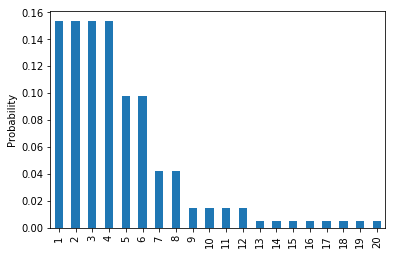# 1. 点数之和

在《龙与地下城》角色扮演游戏中，玩家通过投掷 3 个 6 面骰子来决定自己角色的属性。求投掷 3 个 6 面骰子点数之和的分布。

这个问题可以从通过模拟或枚举的方法来计算。

## 1.1. 模拟

可以通过多次模拟投掷 3 个骰子的过程，并汇总结果，得到结果的分布。定义代表单个骰子的 Die 类如下：

d6 是一个 6 面骰子， dice 是包含 3 个 d6 的列表；sample_sum() 用于从 dice 中进行采样，代码为：

random_sum() 的参数 dists 是一个分布的列表，它依调用每个分布的 random() 方法并对结果求和，这里相当于计算投掷 3 个 6 面骰子得到的点数之和。sample_sum() 则会对 dists 进行 n 次试验，得到一个结果列表，生成一个 Pmf。

## 1.2. 枚举

除了像上面一样通过模拟投掷 3 个 6 面骰子的过程来得到骰子点数和的分布，还可以枚举所有可能的结果并计算其概率。代码如下：

Pmf 重载了加号操作符如下：

__add__() 会计算自身和 other 两个 Pmf 相加，

绘制模拟和枚举的结果：# 2.最大化

假设我们使用这 3 个 6 面骰子连续投掷了 6 轮，记下每一轮中三个骰子点数之和，其最大值的分布是怎样的呢？这个问题依旧可以从模拟和枚举的角度来计算。

模拟的代码如下：

## 2.2. 枚举

定义 pmf_max() 如下：

pmf_max() 用于求两个 Pmf 中最大值得概率，它会遍历 pmf_1pmf_2 中的所有的条目，取较大的值，并更新其概率。

枚举的过程如下：

绘制模拟和枚举的结果：# 2.3. 使用 CDF 计算最大值的分布

前面模拟和枚举的方法都涉及大量迭代，计算速度较慢。可以使用 CDF 来加速计算。由 CDF 的定义，有：

\begin{equation}
CDF(x) = P(X \leq x)
\end{equation}

设 $X$ 的 CDF 为 $CDF_X$，$Y$ 的 CDF 为 $CDF_Y$，现从 $X$ 中选出一个值 $x$、从 $Y$ 中选出一个值 $y$，计算最大值 $z = max(x, y)$，对于一个任意值 $z_0$，若要 $z \leq z_0$，则要求 $x \leq z_0$ 且 $y \leq a$。如果 $X$ 和 $Y$ 是独立的，则有：

\begin{equation}
CDF_Z(z) = CDF_X(z)CDF_Y(z)
\end{equation}

如果从 $CDF_X$ 中选出 $k$ 个值 $x_1, x_2,…,x_k$，则 $z = max(x_1, x_2,…,x_k)$ 的 CDF 为：

\begin{equation}
CDF_Z(z) = CDF_X(z)^k
\end{equation}

据此可以在 Cdf 类中实现 max() 方法如下：

回到使用这 3 个 6 面骰子投掷 6 轮求最大值分布的问题，代码如下：# 3. 混合分布

在前面的问题中，我们只使用了 6 面骰子，假设我们有一盒骰子，其中有：

• 2 个 4 面骰子
• 3 个 6 面骰子
• 2 个 8 面骰子
• 1 个 12 面骰子
• 1 个 20 面骰子

现在从盒子中随机选择一个投掷，得到的点数的分布是怎样的呢？

可以使用如下代码构造一个 Pmf，包含各种骰子的 Pmf 以及选中它的概率：

定义 make_mixture() 用于计算混合概率：

从盒子中随机选择一个投掷，得到的点数的分布为：